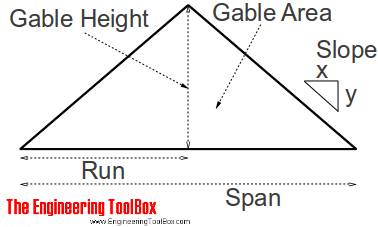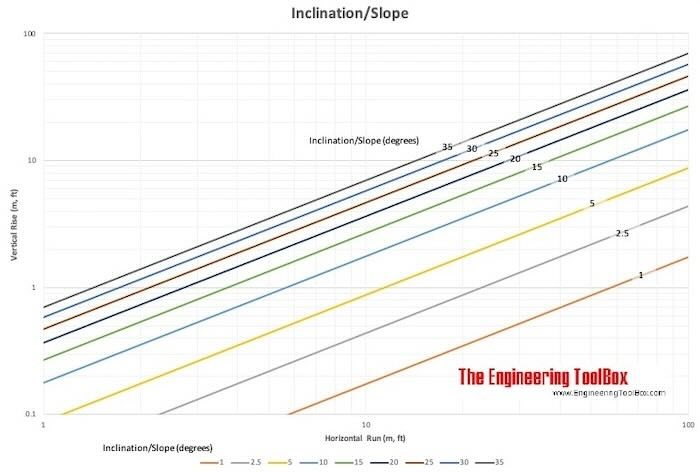Engineering ToolBox - Resources, Tools and Basic Information for Engineering and Design of Technical Applications!

# Roof Framing

## Run, roof slope, gable height and gable area.### Run

Run can be calculated as:

R = S / 2                        (1)

where

R = run (ft, m)

S = span (ft, m)

#### Example - Run

The run of a roof with 60 feet span is

R = (60 feet) / 2

= 30 feet

### Gable Height

Gable height can be calculated as

GH = R VR                       (2)

where

GH = gable height (ft, m)

R = run (ft, m)

VR = vertical rise (in/ft, cm/m)

### Example - Gable Height

The Gable Height of a roof with 30 feet run and vertical rice 6 inches per foot, can be calculated as

GH = (30 feet) (6 inches/foot)

= 180 inches

= 15 feet

### Gable Area

Gable Area can be calculated as

GA = GH R                        (3)

where

GA = gable area (sq.ft, m2)

GH = gable height (ft, m)

R = run (ft, m)

### Roof Slope

Roof slope is the ratio between the vertical rise and the horizontal run:

RS = y / x                      (4)

where

RS = roof slope

y = vertical rise (in, m)

x = horizontal run (in, m)

In the United States, slope is typically given in inches per 1 foot or as a ratio of inches per 12 inches and is often referred to with units of "pitch". Ex.

4 in 12

or

4 pitches, 4:12 pitch

In metric countries it is common to use degrees or 10 or 100 as denominator. Ex.

21.8%

or

4:10## Related Topics

• Miscellaneous - Engineering related topics like Beaufort Wind Scale, CE-marking, drawing standards and more.

## Engineering ToolBox - SketchUp Extension - Online 3D modeling!

Add standard and customized parametric components - like flange beams, lumbers, piping, stairs and more - to your Sketchup model with the Engineering ToolBox - SketchUp Extension - enabled for use with the amazing, fun and free SketchUp Make and SketchUp Pro .Add the Engineering ToolBox extension to your SketchUp from the SketchUp Pro Sketchup Extension Warehouse!

Translate

## Privacy

We don't collect information from our users. Only emails and answers are saved in our archive. Cookies are only used in the browser to improve user experience.

Some of our calculators and applications let you save application data to your local computer. These applications will - due to browser restrictions - send data between your browser and our server. We don't save this data.

## Citation

• Engineering ToolBox, (2010). Roof Framing. [online] Available at: https://www.engineeringtoolbox.com/roof-framing-d_1715.html [Accessed Day Mo. Year].

Modify access date.

. .

#### Scientific Online Calculator3 30